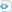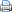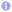C++

# C++11/14 for scientific computing V

## $\boldsymbol{\lambda}$ functions

Anonymous functions, often called $\lambda$ functions, are a common feature of scientific programming languages, e.g., Male and Matlab. They are particularly usefull when functions are employed, which take other functions as arguments.  For example, a numerical root finding algorithm requires to specify a function as an input parameter.

C++11 introduces $\lambda$ functions to C++ and in C++14 they have been extended and become slightly more flexible.  $\lambda$ functions can be assigned to variables similar to functors.  The main advantage of $\lambda$ functions over functors is that they can be defined where needed.  Furthermore, $\lambda$ functions capture variables in the current scope, thus they represent a closure.

The syntax of a $\lambda$ function has basically the form

[ capture-list ] ( params ) -> ret { body }

The possibly empty capture-list specifies which variables are captured and how they are captured (by value or by reference).  Then the arguments and the return type are specified followed by the definition of the function body.  The return type may be omitted if the function body consists of nothing but a single return statement with an expression.

The use of $\lambda$ functions is probably best explained by providing a specific example. In the following, a generic root finding algorithm (regula falsi) is implemented, which looks in an interval for a root of some function.

 Source code#include <iostream>
#include <iomanip>
#include <cstdlib>
#include <cmath>
#include <limits>

template<typename T, typename F>
T regula_falsi(T s, T t, F f) {
typedef enum { none, left, right } side_t;
side_t side(none);
// starting values at endpoints of interval
T fs=f(s);
T ft=f(t);
T r;
for (int n=0; n<std::numeric_limits<T>::digits+2; ++n) {
r=(fs*t - ft*s)/(fs - ft);
if (std::abs(t-s)<std::numeric_limits<T>::epsilon()*std::abs(t+s))
break;
T fr=f(r);
if (fr*ft>0) { // fr and ft have same sign, copy r to t
t=r;
ft=fr;
if (side==left)
fs/=2;
side=left;
} else if (fs*fr>0) { // fr and fs have same sign, copy r to s
s=r;
fs=fr;
if (side==right)
ft/=2;
side=right;
} else { // fr*f_ very small (looks like zero)
break;
}
}
return r;
}

int main() {
// assign lambda function to variable and use afterwards
auto f = [](double x){ return std::cos(x)-x*x*x; };
double x0=regula_falsi(0., 1., f);
// specify lambda function directly as an argument
double x1=regula_falsi(0., 3., [](double x){ return std::cos(x); });
std::cout << std::setprecision(12)
<< x0 << '\n'
<< x1 << '\n';
return 0;
}